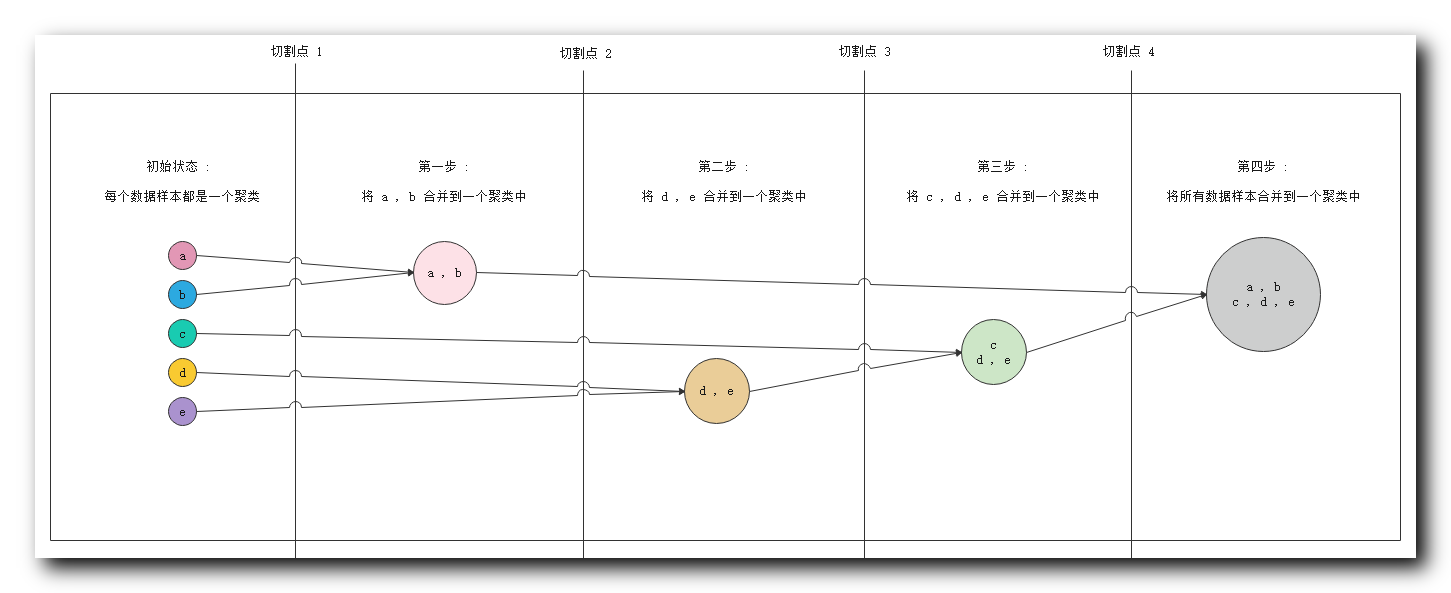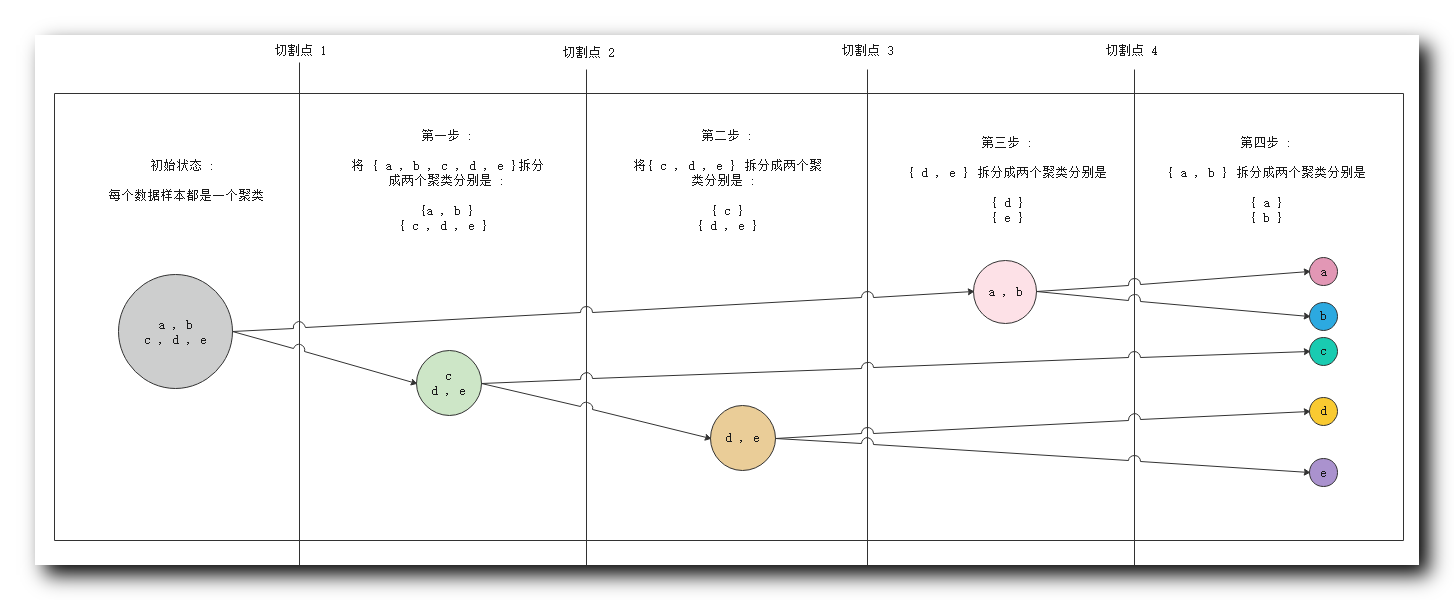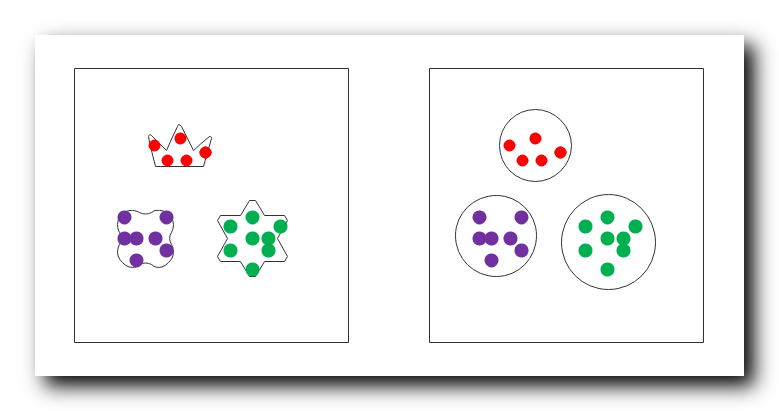• 基于层次的聚类方法 简介 基于层次的聚类方法 概念 聚合层次聚类 图示 划分层次聚类 图示 基于层次的聚类方法 切割点选取 族间距离 概念 族间距离 使用到的变量 族间距离 最小距离 ...基于层次聚类总结
文章目录基于层次的聚类方法 简介基于层次的聚类方法 概念聚合层次聚类 图示划分层次聚类 图示基于层次的聚类方法 切割点选取族间距离 概念族间距离 使用到的变量族间距离 最小距离族间距离 最大距离族间距离 中心点距离族间距离 平均距离基于层次聚类 ( 聚合层次聚类 ) 步骤基于层次聚类 ( 聚合层次聚类 ) 算法终止条件族半径 计算公式基于层次聚类总结

基于层次的聚类方法 简介

1 . 基于层次的聚类方法 : 将 数据集样本对象 排列成 聚类树 , 在 指定 的层次 ( 切割点 ) 进行切割 , 切割点 时刻 的聚类分组 ,  就是 最终需要的聚类分组 ; 也就是这个切割点的切割的时刻 , 互相关联的样本 , 划分到一个聚类分组中 ;

2 . 基于层次聚类方法 的两种方式 :

① 聚合层次聚类 : 开始时 , 每个对象都是一个聚类分组 ( 原子聚类 ) , 根据 聚类之间的相似性 , 对原子聚类逐渐合并 , 最终会合并成一个聚类 ; 其 本质是 由 多个聚类分组 切割成 成少数 聚类分组 ;

② 划分层次聚类 : 开始时 , 所有的样本都在一个聚类中 , 根据聚类间相似性 , 对聚类进行划分 , 最终 每个样本 都会被划分成一个聚类分组 ( 原子聚类 ) ;  本质是 由 少数 聚类分组 划分成多个 聚类分组 ;

基于层次的聚类方法 概念

1 . 基于层次的聚类方法 概念 : 将数 据集样本对象 排列成 树结构 , 称为 聚类树 , 在指定的层次 ( 步骤 ) 上切割数据集样本 , 切割后时刻的 聚类分组 就是 聚类算法的 聚类结果 ;

2 . 基于层次的聚类方法 : 一棵树可以从叶子节点到根节点 , 也可以从根节点到叶子节点 , 基于这两种顺序 , 衍生出两种方法分支 , 分别是 : 聚合层次聚类 ,  划分层次聚类 ;

3 . 聚合层次聚类 ( 叶子节点到根节点 ) : 开始时 , 每个样本对象自己就是一个聚类 , 称为 原子聚类 , 然后根据这些样本之间的 相似性 , 将这些样本对象 ( 原子聚类 ) 进行 合并 ;
常用的聚类算法 : 大多数的基于层次聚类的方法 , 都是 聚合层次聚类 类型的 ; 这些方法从叶子节点到根节点 , 逐步合并的原理相同 ; 区别只是聚类间的相似性计算方式不同 ;

4 . 划分层次聚类 ( 根节点到叶子节点 ) :  开始时 , 整个数据集的样本在一个总的聚类中 , 然后根据样本之间的相似性 , 不停的切割 , 直到完成要求的聚类操作 ;

5 . 算法性能 : 基于层次的聚类方法的时间复杂度为 $O(N^2)$ , 如果处理的样本数量较大 , 性能存在瓶颈 ;

聚合层次聚类 图示

1 . 聚合层次聚类 图示 :① 初始状态 : 最左侧 五个 数据对象 , 每个都是一个聚类 ;
② 第一步 : 分析相似度 , 发现 $a , b$ 相似度很高 , 将 $\{a ,b\}$ 分到一个聚类中 ;
③ 第二步 : 分析相似度 , 发现 $d, e$ 相似度很高 , 将 $\{d, e\}$ 分到一个聚类中 ;
④ 第三步 : 分析相似度 , 发现 $c$ 与 $d,e$ 相似度很高 , 将 $c$ 数据放入 $\{d, e\}$ 聚类中 , 组成 $\{c,d, e\}$ 聚类 ;
⑤ 第四步 : 分析相似度 , 此时要求的相似度很低就可以将不同的样本进行聚类 , 将前几步生成的两个聚类 , 合并成一个聚类 $\{a, b, c, d, e\}$ ;

2 . 切割点说明 : 实际进行聚类分析时 , 不会将所有的步骤走完 , 这里提供四个切割点 , 聚类算法进行聚类时 , 可以在任何一个切割点停止 , 使用当前的聚类分组当做聚类结果 ;

① 切割点 $1$ : 在切割点 $1$ 停止 , 会得到 $5$ 个聚类分组 , $\{a\}$ , $\{b\}$, $\{c\}$, $\{d\}$ , $\{e\}$ ;
② 切割点 $2$ : 在切割点 $2$ 停止 , 会得到 $4$ 个聚类分组 , $\{a, b\}$ , $\{c\}$, $\{d\}$ , $\{e\}$ ;
③ 切割点 $3$ : 在切割点 $3$ 停止 , 会得到 $3$ 个聚类分组 , $\{a, b\}$ , $\{c\}$, $\{d, e\}$ ;
④ 切割点 $4$ : 在切割点 $4$ 停止 , 会得到 $2$ 个聚类分组 ; $\{a, b\}$ , $\{c, d, e\}$ ;
⑤ 走完整个流程 : 会得到 $1$ 个聚类分组 ,  $\{a, b ,c, d, e\}$ ;

划分层次聚类 图示

1 . 划分层次聚类 图示 :① 初始状态 : 最左侧 五个 数据对象 , 属于一个聚类 ;
② 第一步 : 分析相似度 , 切割聚类 , 将 $\{c,d, e\}$ 与 $\{a ,b\}$ 划分成两个聚类 ;
③ 第二步 : 分析相似度 , 将 $\{c,d, e\}$ 中的 $\{c\}$ 与 $\{d, e\}$ 划分成两个聚类 ;
④ 第三步 : 分析相似度 , 将 $\{d, e\}$ 拆分成 $\{d\}$ 和 $\{e\}$ 两个聚类 ;
⑤ 第四步 : 分析相似度 , 将 $\{a ,b\}$ 拆分成 $\{a\}$ 和 $\{b\}$ 两个聚类 , 至此所有的数据对象都划分成了单独的聚类 ;

2 . 切割点说明 : 实际进行聚类分析时 , 不会将所有的步骤走完 , 这里提供四个切割点 , 聚类算法进行聚类时 , 可以在任何一个切割点停止 , 使用当前的聚类分组当做聚类结果 ;

① 切割点 $1$ : 在切割点 $1$ 停止 , 会得到 $1$ 个聚类分组 ,  $\{a, b ,c, d, e\}$ ;
② 切割点 $2$ : 在切割点 $2$ 停止 , 会得到 $2$ 个聚类分组 ; $\{a, b\}$ , $\{c, d, e\}$ ;
③ 切割点 $3$ : 在切割点 $3$ 停止 , 会得到 $3$ 个聚类分组 , $\{a, b\}$ , $\{c\}$, $\{d, e\}$$; ④ 切割点 $4$ : 在切割点 $4$ 停止 , 会得到 $4$ 个聚类分组 , $\{a, b\}$ , $\{c\}$, $\{d\}$ , $\{e\}$ ; ⑤ 走完整个流程 : 会得到 $5$ 个聚类分组 , $\{a\}$ , $\{b\}$, $\{c\}$, $\{d\}$ , $\{e\}$ ; 基于层次的聚类方法 切割点选取 1 . 算法终止条件 ( 切割点 ) : 用户可以指定聚类操作的算法终止条件 , 即上面图示中的切割点 , 如 : ① 聚类的最低个数 : 聚合层次聚类中 , $n$ 个样本 , 开始有 $n$ 个聚类 , 逐步合并 , 聚类个数逐渐减少 , 当聚类个数达到最低值 $min$ , 停止聚类算法 ; ② 聚类最高个数 : 划分层次聚类中 , $n$ 个样本 , 开始有 $1$ 个聚类 , 逐步划分 , 聚类个数逐渐增加 , 当聚类个数达到最大值 $max$ , 停止聚类算法 ; ③ 聚类样本的最低半径 : 聚类的数据样本范围不能无限扩大 , 指定一个阈值 , 只有将该阈值内的样本放入一组 ; 半径指的是所有对象距离其平均点的距离 ; 2 . 切割点回退问题 : 切割点一旦确定 , 便无法回退 ; 这里以聚合层次聚类为例 : ① 处于切割点 $4$ : 如已经执行到了步骤三 , 此时处于切割点 $4$ , 聚类分组为 $\{a, b\}$ , $\{c, d, e\}$ ; ② 试图回退到 切割点 $3$ : 想要会回退到切割点 $3$ 的状态 , 视图将聚类分组恢复成 $\{a, b\}$ , $\{c\}$, $\{d, e\}$ ; ③ 无法回退 : 该操作是无法实现的 , 聚类分组一旦 合并 或 分裂 , 此时就无法回退 ; 族间距离 概念 族间距离 : ① 作用: 族间距离 , 就是聚类分组之间的距离 , 之前的距离计算都是 样本 之间的距离 , 这里的基于层次聚类时 , 不管是聚合层次聚类 , 还是划分层次聚类 , 其都要进行 聚类分组 间的相似度比较 , ② 聚合层次聚类 : 是 根据 聚类的族间距离 ( 聚类分组相似性 ) 将不同的聚类分组进行合并 ; ③ 划分层次聚类 : 是 根据 聚类的族间距离 ( 聚类分组相似性 ) 将不同的聚类分组进行划分 ( 拆分 ) ; 族间距离 使用到的变量 公式中 用到的 变量 : ① 样本表示 : $p$ 和 $q$ 表示 分别 处于两个聚类分组中的 两个样本 ; ② 样本距离表示 : $d(p, q)$ 表示 $p$ 样本对象 与 $q$ 样本对象的距离 ; ③ 聚类 ( 族 ) 表示 : $C_i$ 和 $C_j$ 分别表示两个 聚类 / 族 / 聚类分组 ; ④ 聚类距离表示 : $d(C_i, C_j)$ 表示 $C_i$ 聚类 与 $C_j$ 聚类 之间的距离 ; ⑤ 聚类中心点 : $m_i$ 是 $C_i$ 聚类的中心点 , $m_j$ 是 $C_j$ 聚类的中心点 ; ⑥ 样本个数 : $n_i$ 是 $C_i$ 聚类的样本个数 , $n_j$ 是 $C_j$ 聚类的样本个数 ; 族间距离 最小距离 $C_i \,, C_j$ 族间距离 最小距离 公式 : $d_{min}(C_i , C_j) = min _{p \in C_i , q \in C_j} d(p, q)$ $d_{min}(C_i , C_j)$ 表示两个聚类的最小距离 ; $p$ 是属于 $C_i$ 聚类中的任意样本 ; $q$ 是属于 $C_j$ 聚类中的任意样本 ; 总结 : 两个聚类中两个最近的样本之间的距离就是 聚类间的 最小距离 ; 族间距离 最大距离 $C_i \,, C_j$ 族间距离 最大距离 公式 : $d_{max }(C_i , C_j) = max _{p \in C_i , q \in C_j} d(p, q)$ $d_{max }(C_i , C_j)$ 表示两个聚类的最大距离 ; $p$ 是属于 $C_i$ 聚类中的任意样本 ; $q$ 是属于 $C_j$ 聚类中的任意样本 ; 总结 : 两个聚类中两个最远的样本之间的距离就是 聚类间的 最大距离 ; 族间距离 中心点距离 $C_i \,, C_j$ 族间距离 中心点距离 公式 : $d_{mean }(C_i , C_j) = d(m_i, m_j)$ $d_{mean }(C_i , C_j)$ 表示两个聚类的 中心点距离 ; $m_i$ 是 $C_i$ 聚类的中心点 ; $m_j$ 是 $C_j$ 聚类的中心点 ; $d(m_i, m_j)$ 表示 $m_i$ 样本 和 $m_j$ 样本 之间的距离 ; 总结 : 两个聚类中的中心点样本之间的距离就是 聚类间的 中心点距离 ; 族间距离 平均距离 $C_i \,, C_j$ 族间距离 平均距离 公式 : $d_{avg}(C_i , C_j) = \frac{1}{n_i n_j}\sum_{p \in C_i}\sum_{q \in C_j} d(p, q)$ $d_{mean }(C_i , C_j)$ 表示两个聚类的 中心点距离 ; $p$ 是属于 $C_i$ 聚类中的任意样本 ; $q$ 是属于 $C_j$ 聚类中的任意样本 ; $n_i$ 是 $C_i$ 聚类的样本个数 ; $n_j$ 是 $C_j$ 聚类的样本个数 ; $\sum_{p \in C_i}\sum_{q \in C_j} d(p, q)$ 表示 聚类 $C_i$ 中每一个点 到 聚类 $C_j$ 中所有点的距离 , 这里 $C_i$ 中每个点都对应 $n_j$ 个距离 , $n_i$ 个点 , 对应 $n_i \times n_j$ 个距离 ; 总结 : 两个聚类中的 平均距离 就是 聚类间的 所有点的距离的平均距离 ; 基于层次聚类 ( 聚合层次聚类 ) 步骤 聚合层次聚类步骤 : ① 原理 : 根据 聚类分组 的 族间距离 对相似的 聚类分组 进行 逐步合并 ; ② 步骤一 : 每个样本都构成 聚类分组 , 称为 原子聚类 ; ③ 步骤二 : 计算所有 聚类 之间的距离 ; 可以采用 最小距离 , 最大距离 , 中心点距离 , 平均距离 中的一个 ; ④ 步骤三 : 将距离最近的两个 聚类分组 合并 , 聚类的个数 减少 $1$ 个 ; ⑤ 步骤四 : 转到 步骤二 计算聚类间距离 , 步骤三 合并近距离聚类 ; 如果满足算法终止条件 , 那么停止聚类 , 否则一直循环迭代 , 最终合并成一个聚类 ; 基于层次聚类 ( 聚合层次聚类 ) 算法终止条件 算法终止条件 : 是由 用户 指定的 , 如 : ① 聚类分组 ( 族 ) 个数 : 当聚类的个数达到阈值 , 算法终止 ; ② 聚类半径 : 每个 聚类的半径 都超过某个阈值 ; 族半径 计算公式 族 ( 聚类 ) 半径计算公式 : $R=\frac{1}{n}\sum _{i=1}^n d(p_i - m)$ $R$ 表示聚类半径 ; $n$ 表示聚类中的 样本 个数 ; $m$ 代表聚类中心点 ; $d(p_i - m)$ 表示聚类中第 $i$ 个样本距离中心点的距离 ; 基于层次聚类总结 1 . 基于层次聚类 的核心 : 是计算 两个 聚类分组 ( 族 ) 之间的距离 , 根据 族间距离 进行 聚类合并 ; 2 . 适用场景 : 如果 每个 聚类 密度差不多 , 族间距离 分离的很清晰 , 那么使用不同的 族间距离 进行聚类 产生的聚类结果 基本一致 ; 3 . 算法缺陷 : 基于层次距离不适用于以下情况 ; 聚类分组 分离的不明显 ; 形状不是球形 , 凹形的 ; 聚类间大小不等 ; 各个聚类间样本密度不同 ;  展开全文聚类 数据挖掘 机器学习 •研究论文 •研究论文 •研究论文 •研究论文 •研究论文 • 多字典学习的图像超分辨率重建过程中常见的K均值聚类、高斯混合模型聚类等方法会导致图像的重建质量欠佳且不稳定，针对这一问题提出一种新的基于层次聚类的图像超分辨率重建算法；首先对样本图像块提取特征并进行... • 针对自然图像分割中,由于单一的颜色空间难以表示复杂多变的场景信息以及目标与背景的低对比度等产生的过分割和误分割问题,提出了选择RGB、XYZ和LUV这3个颜色空间的增强图像进行基于层次聚类的融合分割的新方法。... • 目的 利用层次聚类与人工免疫模式识别相结合的方法解决无监督结构健康监测中对结构故障识别和分类的问题．方法 通过凝聚型层次聚类实现样本...结论 基于层次聚类和人工免疫的无监督结构故障检测与分类算法通过免疫学 • 提出了一种基于层次聚类（HCCG）的复杂网络粗粒度方法。 通过使用层次聚类方法对网络节点进行分组，然后更新聚类之间边缘的权重以提取粗粒度网络。 在几个典型的复杂网络上进行的大量仿真实验表明，HCCG方法可以... • 基于层次聚类及因子分析的跨地域农村居民消费研究，白媛，李剑英，在研究者对新农村全面小康评价体系的基础上，针对其中的农村居民生活消费支出一项，对部分地区农村居民消费情况进行分析及预测。 • 基于层次聚类的多维数据分析算法，凝聚层次算法: 初始阶段，将每个样本点分别当做其类簇，然后合并这些原子类簇直至达到预期的类簇数或者其他终止条件。 传统的凝聚层次聚类算法有AGENES，初始时，AGENES将每个样本... • 针对现有的孤立点检测算法在通用性、有效性、用户友好性及处理高维大数据集的性能还不完善，提出了一种快速有效的基于层次聚类的全局孤立点检测方法。该方法基于层次聚类的结果，根据聚类树和距离矩阵可视化判断数据...孤立点检测 数据挖掘 • 基于层次聚类的数码复印机日志审计研究，张霖，李祺，本文从数码复印机审计的角度出发，对复印机留存日志进行聚类分析。针对复印机日志结构特点，对日志进行清洗、序列化，将聚类单位 • 基于案例推理技术在众多领域已经得到了广泛地应用。...分析了案例库层次聚类规则，重点论述了在聚类基础上的案例检索策略。根据实验结果表明，该方法能够有效地提高案例库中案例的利用率以及案例检索的成功率。 • 一个基于层次聚类的二手车估值方法，张德斌，朱郑州，随着二手车市场的扩大，如何突破人工估值速度慢、准确性低的瓶颈，在保证二手车估值高准确度的前提下提高估值速度，是亟待解决的 • 一种基于层次聚类的复杂网络社区划分算法，朱帅兵，殷传涛，随着互联网的发展和普及，人们对复杂网络的研究越来越多。复杂网络中存在社区结构，而找出社区结构有助于挖掘复杂网络中一些有用社区划分 • 首先，利用层次聚类算法对灰度图像进行边缘检测，然后利用区域生长和形态学处理进行图像分割，从而确定二氧化硅颗粒的质心位置坐标，绘制运动轨迹.其次，选取面积和广义半径作为边缘轮廓特征的指标.实验结果表明，... • 属性约简是粗糙集用于...利用自底向上的聚合层次聚类方法对决策表的条件属性集进行聚类,得到条件属性集的k个划分,然后对这k个属性子集进行后处理操作而得到k个有较大差异的约简属性集。实验结果表明了算法的有效性。 • 针对机场噪声数据集特征,提出一种基于代表点的快速层次聚类算法,该算法在传统凝聚层次聚类算法的基础上,借助聚类代表点法和二分法策略进行改进。同时,提出一种聚类代表点和聚类算法相似性定义相结合的聚类结果评价... • 首先运用对称不确定性的概念选择出最相关的流测度，然后利用核函数定理对选择的网络流测度进行高维映射，以测度的高维空间距离作为度量各个类差别的标准，提高了聚类结果的准确性。采用光滑因子、轮廓系数和不确定熵... • 以图书馆为研究对象,根据调查所得的数据,运用统计学方法分析出图书馆借阅情况与高校发展之间的潜在关系。...利用层次聚类对重庆市高校图书馆进行了分类,其结果表明不同类别学校的图书馆存在较大差异。 • (19)中华人民共和国国家知识产权局 (12)发明专利申请 (10)申请公布号 CN 111373395 A (43)申请公布日 2020.07.03 (21)申请号 201880043921.2 (51)Int.Cl. G06F 16/906(2006.01) (22)申请日 2018.08.31 (85)PCT国际... • 运用中文自然语言处理和信息抽取系统识别命名实体和实体关系，生成实体信息对象（Entity Profile），采用实体信息对象（EP）中的个人信息特征，实体关系和上下文相关信息在Hadoop平台上基于凝聚的层次聚类方法解决了... • 案例分析：人人网的基于层次聚类的好友推荐 Case I: Friend recommendation by agglomerative hierarchical clustering – User familiarity• Common friends• User profile• User access• User ...  案例分析：人人网的基于层次聚类的好友推荐 Case I: Friend recommendation by agglomerative hierarchical clustering – User familiarity• Common friends• User profile• User access• User interestHierarchy• Clustering to find communities in social network– All in one community share some properties.– These overlapping communities reveal some social relationship of different levels.– They help to building new friendships in the social network.posted on 2011-12-27 10:42 wentingtu 阅读(...) 评论(...) 编辑 收藏 转载于:https://www.cnblogs.com/wentingtu/archive/2011/12/27/2303129.html 展开全文• 为了改善Java源程序的质量，使之尽可能地符合“高内聚、低耦合”的设计要求，提出了扩展的Jaccard系数，并将其作为实体间相似性的度量公式，采用层次聚类算法将Java类图划分为若干个候选包。在基于交互及度量重构... • 现有的孤立点检测算法在通用性、有效性、用户友好性及处理高维大数据集的性能还不完善，为此，提出一种有效的全局孤立点检测方法，该方法进行凝聚层次聚类，根据聚类树和距离矩阵来可视化判断数据孤立程度，确定孤立... • I . 聚类主要算法 II .... 基于层次的聚类方法 IV . 聚合层次聚类 图示 V . 划分层次聚类 图示 VI . 基于层次的聚类方法 切割点选取 VII . 基于密度的方法 VIII . 基于方格的方法 IX . 基于模型的方法 文章目录I . 聚类主要算法II . 基于划分的聚类方法III . 基于层次的聚类方法IV . 聚合层次聚类 图示V . 划分层次聚类 图示VI . 基于层次的聚类方法 切割点选取VII . 基于密度的方法VIII . 基于方格的方法IX . 基于模型的方法 I . 聚类主要算法 聚类主要算法 : ① 基于划分的聚类方法 : K-Means 方法 ; ② 基于层次的聚类方法 : Birch ; ③ 基于密度的聚类方法 : DBSCAN ( Density-Based Spatial Clustering of Applications with Noise ) ; ④ 基于方格的方法 ; ⑤ 基于模型的方法 : GMM 高斯混合模型 ; II . 基于划分的聚类方法 基于划分的方法 简介 : 基于划分的方法 , 又叫基于距离的方法 , 基于相似度的方法 ; ① 概念 : 给定 $n$ 个数据样本 , 使用划分方法 , 将数据构建成 $k$ 个划分 $(k \leq n)$ , 每个划分代表一个聚类 ; ② 分组 : 将数据集 分成 $k$ 组 , 每个分组至少要有一个样本 ; ③ 分组与样本 对应关系 : 每个分组有 $1$ 个或多个样本对象 ( $1$ 对多 ) , 每个对象同时只能在 $1$ 个分组中 ( $1$ 对 $1$ ) ; ④ 硬聚类 与 软聚类 : 每个数据对象只能属于一个组 , 这种分组称为硬聚类 ; 软聚类每个对象可以属于不同的组 ; III . 基于层次的聚类方法 1 . 基于层次的聚类方法 概念 : 将数 据集样本对象 排列成 树结构 , 称为 聚类树 , 在指定的层次 ( 步骤 ) 上切割数据集样本 , 切割后时刻的 聚类分组 就是 聚类算法的 聚类结果 ; 2 . 基于层次的聚类方法 : 一棵树可以从叶子节点到根节点 , 也可以从根节点到叶子节点 , 基于这两种顺序 , 衍生出两种方法分支 , 分别是 : 聚合层次聚类 , 划分层次聚类 ; 3 . 聚合层次聚类 ( 叶子节点到根节点 ) : 开始时 , 每个样本对象自己就是一个聚类 , 称为 原子聚类 , 然后根据这些样本之间的 相似性 , 将这些样本对象 ( 原子聚类 ) 进行 合并 ; 常用的聚类算法 : 大多数的基于层次聚类的方法 , 都是 聚合层次聚类 类型的 ; 这些方法从叶子节点到根节点 , 逐步合并的原理相同 ; 区别只是聚类间的相似性计算方式不同 ; 4 . 划分层次聚类 ( 根节点到叶子节点 ) : 开始时 , 整个数据集的样本在一个总的聚类中 , 然后根据样本之间的相似性 , 不停的切割 , 直到完成要求的聚类操作 ; 5 . 算法性能 : 基于层次的聚类方法的时间复杂度为 $O(N^2)$ , 如果处理的样本数量较大 , 性能存在瓶颈 ; IV . 聚合层次聚类 图示 1 . 聚合层次聚类 图示 :① 初始状态 : 最左侧 五个 数据对象 , 每个都是一个聚类 ; ② 第一步 : 分析相似度 , 发现 $a , b$ 相似度很高 , 将 $\{a ,b\}$ 分到一个聚类中 ; ③ 第二步 : 分析相似度 , 发现 $d, e$ 相似度很高 , 将 $\{d, e\}$ 分到一个聚类中 ; ④ 第三步 : 分析相似度 , 发现 $c$ 与 $d,e$ 相似度很高 , 将 $c$ 数据放入 $\{d, e\}$ 聚类中 , 组成 $\{c,d, e\}$ 聚类 ; ⑤ 第四步 : 分析相似度 , 此时要求的相似度很低就可以将不同的样本进行聚类 , 将前几步生成的两个聚类 , 合并成一个聚类 $\{a, b, c, d, e\}$ ; 2 . 切割点说明 : 实际进行聚类分析时 , 不会将所有的步骤走完 , 这里提供四个切割点 , 聚类算法进行聚类时 , 可以在任何一个切割点停止 , 使用当前的聚类分组当做聚类结果 ; ① 切割点 $1$ : 在切割点 $1$ 停止 , 会得到 $5$ 个聚类分组 , $\{a\}$ , $\{b\}$, $\{c\}$, $\{d\}$ , $\{e\}$ ; ② 切割点 $2$ : 在切割点 $2$ 停止 , 会得到 $4$ 个聚类分组 , $\{a, b\}$ , $\{c\}$, $\{d\}$ , $\{e\}$ ; ③ 切割点 $3$ : 在切割点 $3$ 停止 , 会得到 $3$ 个聚类分组 , $\{a, b\}$ , $\{c\}$, $\{d, e\}$ ; ④ 切割点 $4$ : 在切割点 $4$ 停止 , 会得到 $2$ 个聚类分组 ; $\{a, b\}$ , $\{c, d, e\}$ ; ⑤ 走完整个流程 : 会得到 $1$ 个聚类分组 , $\{a, b ,c, d, e\}$ ; V . 划分层次聚类 图示 1 . 划分层次聚类 图示 :① 初始状态 : 最左侧 五个 数据对象 , 属于一个聚类 ; ② 第一步 : 分析相似度 , 切割聚类 , 将 $\{c,d, e\}$ 与 $\{a ,b\}$ 划分成两个聚类 ; ③ 第二步 : 分析相似度 , 将 $\{c,d, e\}$ 中的 $\{c\}$ 与 $\{d, e\}$ 划分成两个聚类 ; ④ 第三步 : 分析相似度 , 将 $\{d, e\}$ 拆分成 $\{d\}$ 和 $\{e\}$ 两个聚类 ; ⑤ 第四步 : 分析相似度 , 将 $\{a ,b\}$ 拆分成 $\{a\}$ 和 $\{b\}$ 两个聚类 , 至此所有的数据对象都划分成了单独的聚类 ; 2 . 切割点说明 : 实际进行聚类分析时 , 不会将所有的步骤走完 , 这里提供四个切割点 , 聚类算法进行聚类时 , 可以在任何一个切割点停止 , 使用当前的聚类分组当做聚类结果 ; ① 切割点 $1$ : 在切割点 $1$ 停止 , 会得到 $1$ 个聚类分组 , $\{a, b ,c, d, e\}$ ; ② 切割点 $2$ : 在切割点 $2$ 停止 , 会得到 $2$ 个聚类分组 ; $\{a, b\}$ , $\{c, d, e\}$ ; ③ 切割点 $3$ : 在切割点 $3$ 停止 , 会得到 $3$ 个聚类分组 , $\{a, b\}$ , $\{c\}$, $\{d, e\}$$ ;
④ 切割点 $4$ : 在切割点 $4$ 停止 , 会得到 $4$ 个聚类分组 , $\{a, b\}$ , $\{c\}$, $\{d\}$ , $\{e\}$ ;
⑤ 走完整个流程 : 会得到 $5$ 个聚类分组 , $\{a\}$ , $\{b\}$, $\{c\}$, $\{d\}$ , $\{e\}$ ;

VI . 基于层次的聚类方法 切割点选取

1 . 算法终止条件 ( 切割点 ) : 用户可以指定聚类操作的算法终止条件 , 即上面图示中的切割点 , 如 :

① 聚类的最低个数 :  聚合层次聚类中 , $n$ 个样本 , 开始有 $n$ 个聚类 , 逐步合并 , 聚类个数逐渐减少 , 当聚类个数达到最低值 $min$ , 停止聚类算法 ;
② 聚类最高个数 : 划分层次聚类中 , $n$ 个样本 , 开始有 $1$ 个聚类 , 逐步划分 , 聚类个数逐渐增加 , 当聚类个数达到最大值 $max$ , 停止聚类算法 ;
③ 聚类样本的最低半径 : 聚类的数据样本范围不能无限扩大 , 指定一个阈值 , 只有将该阈值内的样本放入一组 ; 半径指的是所有对象距离其平均点的距离 ;

2 . 切割点回退问题 : 切割点一旦确定 , 便无法回退 ; 这里以聚合层次聚类为例 :

① 处于切割点 $4$ : 如已经执行到了步骤三 , 此时处于切割点 $4$ ,  聚类分组为 $\{a, b\}$ , $\{c, d, e\}$ ;
② 试图回退到 切割点 $3$ : 想要会回退到切割点 $3$ 的状态 , 视图将聚类分组恢复成 $\{a, b\}$ , $\{c\}$, $\{d, e\}$ ;
③ 无法回退 : 该操作是无法实现的 , 聚类分组一旦 合并 或 分裂 , 此时就无法回退 ;

VII . 基于密度的方法

1 . 基于距离聚类的缺陷 : 很多的聚类方法 , 都是 基于样本对象之间的距离 ( 相似度 ) 进行的 , 这种方法对于任意形状的分组 , 就无法识别了 , 如下图左侧的聚类模式 ;  这种情况下可以使用基于密度的方法进行聚类操作 ;
基于距离的方法 , 是基于欧几里得距离函数得来 , 其基本的形状都是球状 , 或凸形状 , 如下图右侧的形状 ; 无法计算出凹形状 , 如下图左侧的形状 ;2 . 基于密度的聚类方法 : 相邻的区域内 样本对象 的密度超过某个阈值 , 聚类算法就继续执行 , 如果周围区域密度都很小 , 那么停止聚类方法 ;

① 密度 : 某 单位大小 区域内的样本对象个数 ;
② 聚类分组要求 : 在聚类分组中 , 每个分组的数据样本密度都 必须达到密度要求的最低阈值 ;

3 . 基于密度的聚类方法 算法优点 :

① 排除干扰 :  过滤噪音数据 , 即密度很小 , 样本分布稀疏的数据 ;
② 增加聚类模式复杂度 : 聚类算法可以识别任意形状的分布模式 , 如上图左侧的聚类分组模式 ;

VIII . 基于方格的方法

1 . 基于方格的方法 : 将数据空间划分成 一个个方格 , 在这些方格数据结构上 , 将每个方格中的数据样本 , 当做一个数据处理 , 进行聚类操作 ;

2 . 基于方格的方法优点 : 处理速度很快 , 将每个方格都作为一个数据 , 如果分成 少数的几个方格进行聚类操作 , 聚类瞬间完成 ; 其速度与数据集样本个数无关 , 与划分的数据方格个数有关 ;

3 . 局限性 : 该方法的错误率很高 ;

IX . 基于模型的方法

基于模型的方法

① 基于统计的方法  : GMM 高斯混合模型 ;
② 神经网络方法 ;


展开全文...